# HC Verma Solutions Class 11 Chapter 3 Rest and Motion Kinematics

HC Verma Class 11 Solutions for Chapter 3 Rest and Motion kinematics is one of the important chapters included in the HC Verma book. The chapter mainly deals with different problem sets especially involving the calculation of certain distances, along with other different topics such as uniform velocity, acceleration, displacement, average acceleration, speed, distance and deceleration, etc. These are some of the various metrics that are introduced in the chapter. By preparing from the solutions that we have provided, students can study more effectively and be well equipped to face major exams such as the JEE main exam. Students can refer the HC Verma solutions for Class 11 physics chapter 3 – Rest and Motion: Kinematics to gain a much deeper understanding of the concepts. This can be incredibly beneficial in making students become more adept and proficient in solving problems.

Students will learn about topics like;

1. Rest and Motion
2. Distance and Displacement
3. Average Speed and Instantaneous Speed
4. Average Velocity and Instantaneous Velocity
5. Average Acceleration and Instantaneous Acceleration
6. Motion in a Straight Line
7. Motion in a Plane
8. Projectile Motion
9. Change of Frame

## Class 11 Important Questions In Chapter 3

1. If a moving particle has a constant velocity then its instantaneous velocity, speed and average velocity are all equal. What are your thoughts about this statement?
2. Can you name some instances where (i) the velocity is perpendicular to the acceleration (ii) the velocity of a particle is zero but its acceleration is not zero, (iii) the velocity is opposite in direction to the acceleration?
3. Can you tell at which point a projectile will have the lowest speed?
4. If two guns are fired at the same time horizontally and with bullets moving at different speeds. Can you determine which bullet will hit the ground first?
(a) bullet moving with slower speed (b) bullet moving with faster speed (c) both will reach simultaneously (d) depends on the masses.
5. What happens when a particle is accelerating?

## HC Verma Solutions Vol 1 Rest and Motion: Kinematics Chapter 3

Question 1: A man a has to go 50 m due north, 40 m due east and 20 m due south to reach a field.

(a) What distance he has to walk to reach the field?

(b) What is his displacement from his house to the field?

Solution: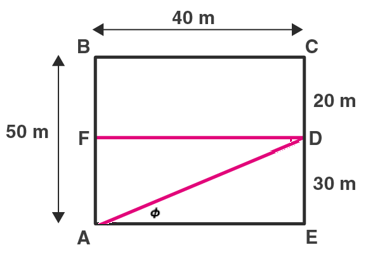(a) Let A be the starting point and D be the field, ending point

Distance that man has to walk to reach the field = AB + BC + CD

= 50 + 40 + 20 = 110

So, man must walk 110 m to reach the field.

(b) Displacement from man’s house to the field, which is AD

AD = √(AE^2 + ED^2) = 50 m

From the triangle AED

tan φ = DE/AE = 30/40 = 3/4

or φ = tan-1(3/4)

Therefore, his displacement from his house to the field is 50 m, tan-1 (3/4) north to east.

Question 2: A particle starts from the origin, goes along the X-axis to the point (20 m, 0) and then returns along the same line to the point (-20 m, 0). Find the distance and displacement of the particle during the trip.

Solution:

Let O be the starting point and coordinates are, O(0, 0), A(20, 0) and B(-20,0)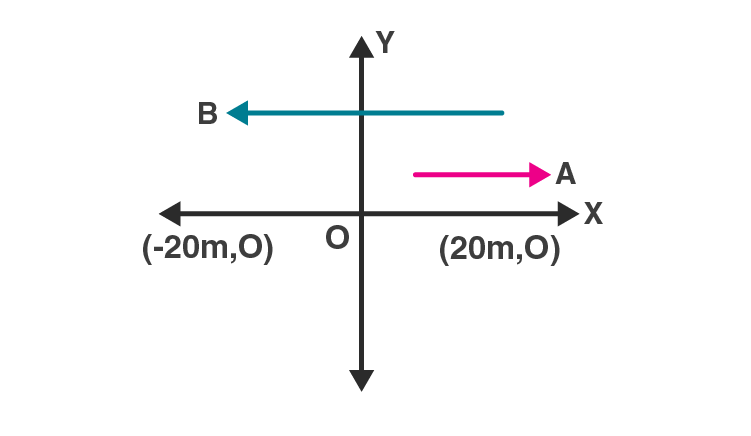Distance travelled = OA + AB = 20m + 40 m = 60 m

Displacement = distance between final and initial point

= OB = 20 m (negative direction)

Question 3: It is 260 km from Patna to Ranchi by air and 320 km by road. An aeroplane takes 30 minutes to go from Patna to Ranchi whereas a deluxe bus takes 8 hours.

(a) Find the average speed of the plane.

(b) Find the average speed of the bus.

(c) Find the average velocity of the plane.

(d) Find the average velocity of the bus.

Solution:

Given:

Total distance travelled by aeroplane from Patna to Ranchi =260 km

Total time taken by aeroplane = 30 min = 0.5 hour

And

Total distance travelled by deluxe bus = 320 km

Total time taken by bus = 8 hour

(a)

Average Speed of the plane = (Total distance travelled)/(Total time taken)

= 260/0.5 = 520 km/h

(b)

Average Speed of the bus = (Total distance travelled)/(Total time taken)

= 320/8

= 40 km/h

(c)

Average velocity of the plane = Displacement/Time

= 260/0.5

= 520 km/h

(d)Average velocity of Bus = Displacement/Time

= 260/8

= 32.5 km/h

Question 4: When a person leaves his home for sightseeing by his car, the meter reads 12352 km. When he returns home after two hours the reading is 12416 km.

(a) What is the average speed of the car during this period?

(b) What is the average velocity?

Solution:

Using formulas:

Average Speed = (Total distance travelled)/(Total time taken)

Average velocity = Displacement/Time

(a) Average speed of the car = (12416-12352)/2 = 32 km/hr

(b) Average velocity = 0/2 = 0

[As person returns to his house, the displacement is zero.]

Question 5: An athlete takes 20 s to reach his maximum speed of 180 km/h. What is the magnitude of his average acceleration?

Solution:

Initial velocity, u = 0

Final velocity, v = 180 km/h = 50 m/s and

Time, t = 20 s

We know, V = u + at

Where V = final velocity, u = initial velocity, a = acceleration and t= time

or a = (V-u)/t = 50/20 = 2.5 m/s2

Question 6: The speed of a car as a function of time is shown in figure (3-E1). Find the distance travelled by the car in 8 seconds and its acceleration.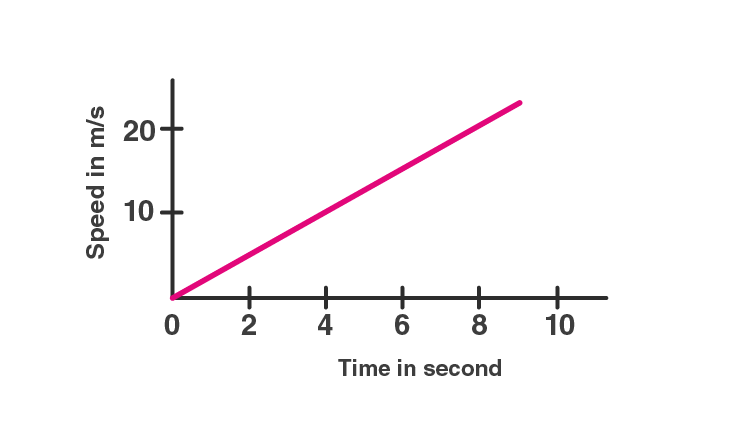Solution:

From the given figure,

The velocity changes from 0 to 20 m/s in the interval of 8 sec.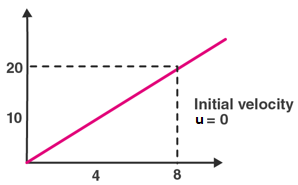So, average acceleration = 20/8 = 2.5 m/s2

Now,

Distance travelled by car, S = ut + at2/2

Here u = 0 (initial velocity)

By substituting values, we get

S = 0 + 1/2 x (2.5) x (82) = 80 m

Question 7: The acceleration of a cart started at t = 0, varies with time as shown in figure (3-E2). Find the distance travelled in 30 seconds and draw the position-time graph.

Solution:

Let u = initial velocity and v = final velocity

In first 10 s, distance travelled, S1 = ut + 1/2 at2

= 0 + 1/2 x 5 x 100 ft

= 250 ft

At 10 sec,

V = u + at

= 0 + 5 x 10

= 50 ft/sec

Therefore, distance traveled from 10s to 20s, it moves with a uniform velocity of 50 ft/sec

Again,

Distance covered from t=10 sec to t=20 sec,

S2 = vt = 50×10 = 500 ft

From figure, acceleration is constant between 20 s and 30 s .i.e -5 ft/m2

At 20 s velocity is 50 ft/s

time, t = 30s – 20 s = 10 s

S3 = ut + 1/2 at2

= 50 x 10 + 1/2 x -5 x (102

= 250 ft

Total distance travelled in 30 s = S1 + S2 + S3 = 250 + 500 + 250 = 1000 ft

Position –Time Graph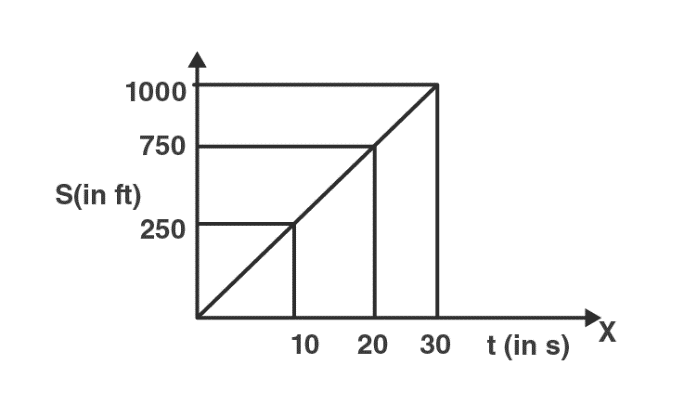Question 8: Figure (3-E3) shows the graph of velocity versus time for a particle going along the X-axis. Find (a) the acceleration,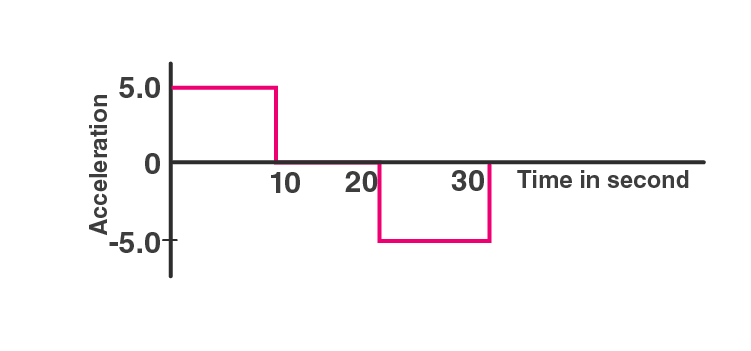(b) the distance travelled in 0 to 10 s and

(c) the displacement in O to 10 s.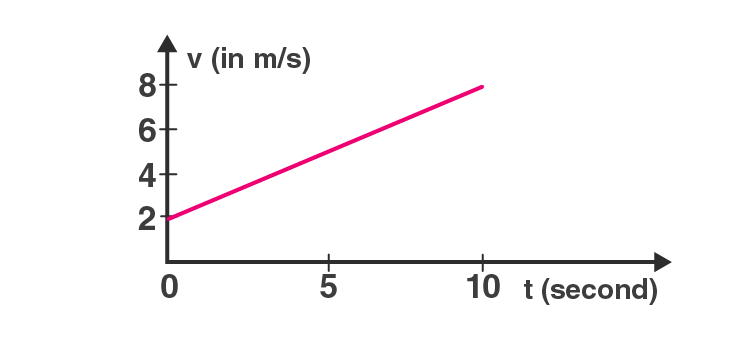Solution:

Initial velocity = u = 2 m/s

Final velocity = V = 8 m/s and

time = t = 10 sec

(a) Acceleration

using formula: V = u + at

i.e. a = (8-2)/10 = 0.6 m/s^2

(b) Again, we know the relation, V2 – u2 = 2aS

or S = 50 m

(c) Here displacement is same as the distance travelled i.e. 50 m

Question 9: Figure (3-E4) shows the graph of the x-coordinate of a particle going along the X-axis as a function of time. Find (a) the average velocity during O to 10 s,

(b) instantaneous velocity at 2, 5, 8 and 12s.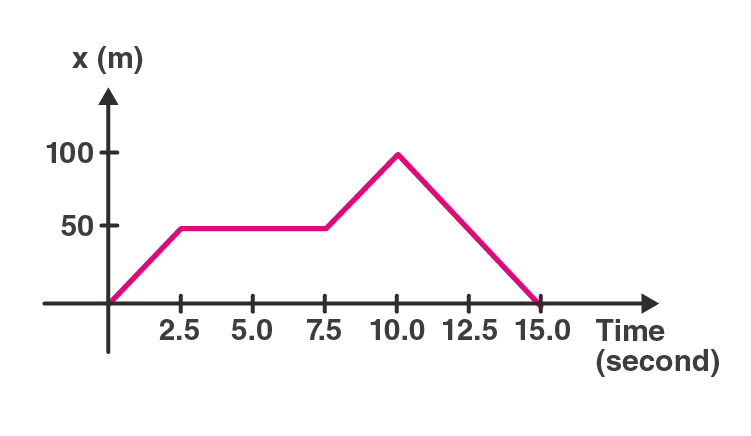Solution:

(a) Displacement from t=0 s to t=10 s is 100 m

Average velocity = Displacement/Time = 100/10 = 10 m/s

(b) Slope of the x-t graph gives velocity,

At t= 2 s, instantaneous velocity = 20 m/s

At t= 5 s, instantaneous velocity = 0 m/s (particle in rest)

At t= 8 s, instantaneous velocity = 20 m/s

At t= 12 s, instantaneous velocity = -20 m/s

[Velocity is negative as it move towards initial position]

Question 10: From the velocity—time plot shown in figure (3-E5), find the distance travelled by the particle during the first 40 seconds. Also find the average velocity during this period.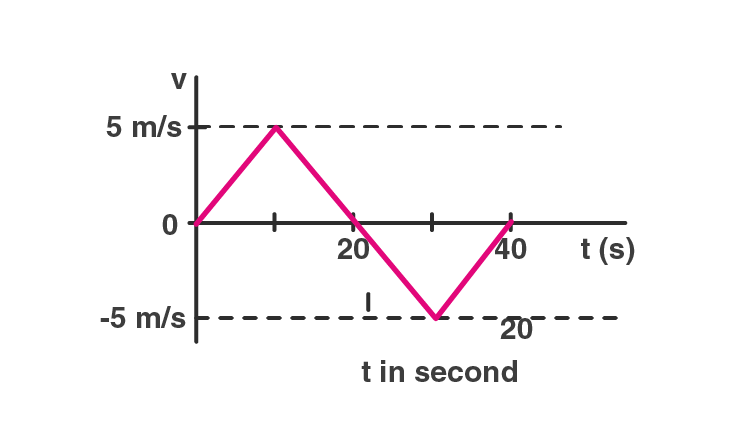Solution:

Area of the V-T graph gives distance travelled

Distance in first 40 sec = Area of triangle OAB+ Area of triangle BCD

= 1/2 x 5 x 20 + 1/2 x 5 x 20

= 100 m

Here the displacement is zero as average velocity is zero.

Question 11: Figure (3-E6) shows x-t graph of a particle. Find the time t such that the average velocity of the particle during the period O to t is zero.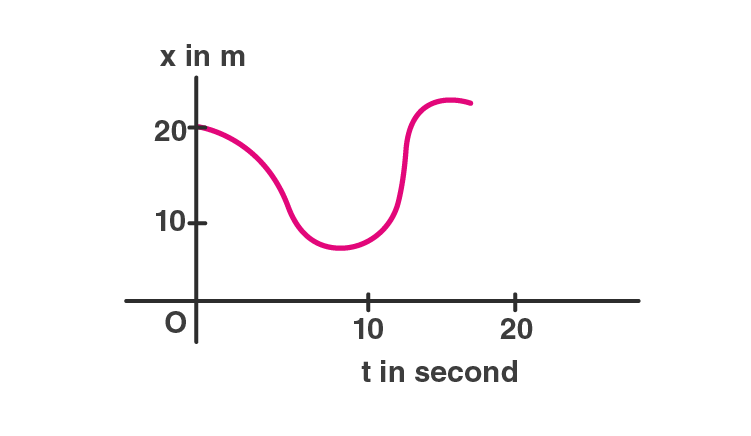Solution:

Let the point B at t = 12 sec

At t = 0, distance = S = 20 m

At t = 12, distance= S = 20 m

From the figure, change in displacement is zero between time interval t=0 to t=12 sec

So. average velocity = 0

Therefore, the average velocity =0 m/s at t=12 sec.

Question 12: A particle starts from a point A and travels along the solid curve shown in figure (3-E7). Find approximately the position B of the particle such that the average velocity between the positions A and B has the same direction as the instantaneous velocity at B.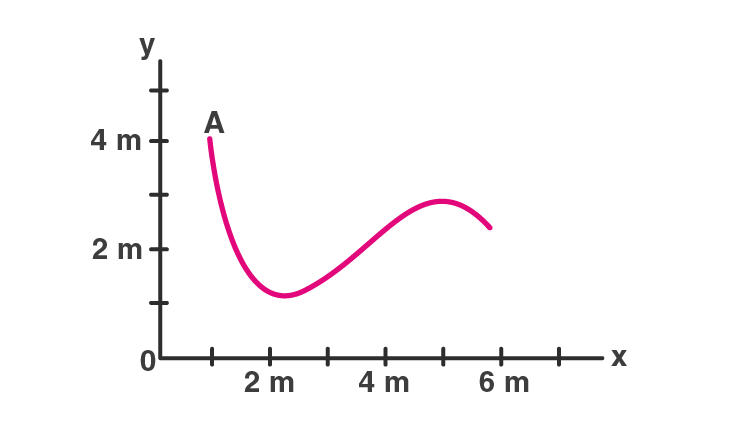Solution: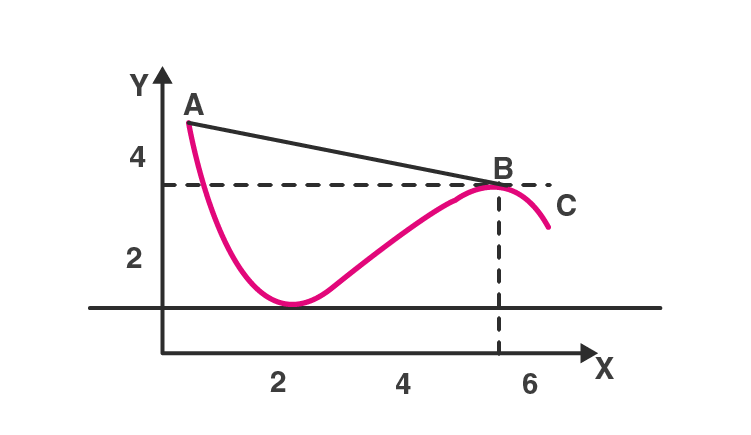At position B, the instantaneous velocity has a direction along BC.

Now, the average velocity between A and B = AB/t

As AB and BC are in same direction, so the point is B (5,3)

Question 13: An object having a velocity 4.0 m/s is accelerated at the rate of 1.2 m/s2 for 5.0 s. Find the distance travelled during the period of acceleration.

Solution:

Initial velocity = u = 4.0 m/s

Time = t = 5.0 s

Acceleration = a = 1.2 m/s2

Let S be the distance travelled, we have

S = ut + 1/2 at2

= 4x 5 + 1/2 x 1.2 x 5 x 5

= 35 m

Question 14: A person travelling at 43.2 km/h applies the brake giving a deceleration of 6.0 m/s2 to his scooter. How far will it travel before stopping?

Solution:

Initial velocity = u = 43.2 km/h = 12 m/s

Final velocity = v = 0 km/h

Acceleration = a = -6.0 m/s2

Using relation, V2 – u2 = 2as

-144= -12 s

or s = 12 m

Hence person will travel 12 m before stopping

Question 15: A train starts from rest and moves with a constant acceleration of 2.0 m/s2 for half a minute. The brakes are then applied and the train comes to rest in one minute. Find (a) the total distance moved by the train, (b) the maximum speed attained by the train and (c) the position(s) of the train at half the maximum speed.

Solution:

Initial velocity = u = 0

Acceleration = a = 2 m/s2

Let V = final velocity before apply breaks

and time=t= 30 sec

Now, V = u + at = 0 + 60 = 60 m/s

(a)

s = ut + 1/2 at2 = 900 m

At u1 = 60 m/s (when break applied)

then v1 = 0 and at t = 60 sec

s1 = ut + 1/2 at2

a1 = (V1-u1)/t = -1 m/s2

and S1 = (V12 – u12)/2a1 = 1800 m

Therefore, total distance travelled by train = s+s1 = 2700 m or 2.7 km

(b) The maximum speed attained by train = v = 60 m/s

(c) Find position of the train

Half the maximum speed = 60/2 m/s = 30 m/s

When acceleration of the train, a=2 m/s2

Distance = S = (V2-u2)/2a = 225 m

When the train is decelerating with, a1=-2 m/s2

then distance = S = (V2-u2)/2a1 = 1350 m

Therefore, Position is 900 + 1350 = 2250 m or 2.25 km from the starting point.

Question 16: A bullet travelling with a velocity of 16 m/s penetrates a tree trunk and Comes to rest in 0.4 m. Find the time taken during the retardation.

Solution:

Initial velocity= u = 16 m/s

Distance covered = s = 0.4 m

Time, t=?

We know acceleration, a = (V2-u2)/2s

= (0-162)/(2×0.4)

= -320 m/s2

Also, t = (V-u)/a

= (0-16)/-320 = 0.05 sec

Question 17: A bullet going with speed 350 m/s enters a concrete wall and penetrates a distance of 5cm before coming to rest. Find the deceleration.

Solution:

Initial velocity = u = 350 m/s

Final velocity = V = 0 m/s

Distance = s = 5 cm = 0.05 m

Now,

a = (V2-u2)/2s

= (0-3502)/(2×0.05) = -12.2 x 105 m/s2

Question 18: A particle starting from rest moves with constant acceleration. If it takes 5.0 s to reach the speed 18.0 km/h find (a) the average velocity during this period, and (b) the distance travelled by the particle during this period.

Solution:

Initial velocity = u = 0 m/s

Final velocity = V = 18 km/h = 5 m/s and

Time = t = 5 sec

Now,

a = (V-u)/t = 5/5 = 1 m/s2

Consider, S be the travelled distance, so

S = ut + 1/2 at2

= 12.5 m

(a) Average velocity = (12.5)/5 = 2.5 m/s

(b) Distance travelled = 12.5 m

Question 19: A driver takes 0.20 s to apply the brakes after he sees a need for it. This is called the reaction time of the driver. If he is driving a car at a speed of 54 km/h and the brakes cause a deceleration of 6.0 m/s2, find the distance travelled by the car after he sees the need to put the brakes on.

Solution:

Given information’s,

Speed of the car = 54 km/hr = 15 m/s (This is the constant speed)

Consider d be the distance travelled while applying brake, then

d = s × t = 15 × 0.2 = 3 m

When driver applied the break,

Initial velocity = u = 15 m/s

Final velocity = v = 0 m/s

Acceleration = a = -6 m/s2

Now,

S = (V2-u2)/2a

= 18.75 m

Total distance = d + s = 3 + 18.75 = 21.75 m or 22 m

Question 20: Complete the following table:

 Car Model Driver X Reaction Time 0.20 s Driver Y Reaction Time 0.30 s A (deceleration on hard breaking = 6.0 m/s2) Speed = 54 km/h Breaking distance a = _____ Total stopping distance b = _____ Speed = 72 km/h Breaking distance c = _____ Total stopping distance d = _____ B (deceleration on hard breaking = 7.5 m/s2) Speed = 54 km/h Breaking distance e = _____ Total stopping distance f = _____ Speed = 72 km/h Breaking distance g = _____ Total stopping distance h = _____

Solution:

Here,

Braking distance means Distance travelled after the brakes are applied.

Total stopping distance = Braking distance + Distance travelled in the reaction time

For Car Model A:

Deceleration = 6.0 m/s2

F driver X:

Initial velocity= u = 54 km/h = 15 m/s

Final velocity = v = 0

Braking distance,

Using relation: a = (V2-u2)/2s = 19 m

Distance travelled in the reaction time = 15 × 0.20 = 3 m

Total stopping distance, b = 19 + 3 = 22 m

For driver Y:

Initial velocity= u = 72 km/h = 20 m/s

Final velocity = v = 0

Braking distance,

c = (V2-u2)/2s = 33m

Distance travelled in the reaction time = 20 × 0.30 = 6 m

Total stopping distance, d = 33 + 6 = 39 m

For Car Model B:

Deceleration = 6.0 m/s2

Similarly, we have e = 15 m

Therefore,

f = 18 m

g = 27 m

and h = 33 m

a = 19m

b= 22 m

c= 33 m

d= 39 m

e = 15 m

f = 18 m

g = 27 m

and h = 33 m

Question 21: A police jeep is chasing a culprit going on a motorbike. The motorbike crosses a turning at a speed of 72 km/h. The jeep follows it at a speed of 90 km/h, crossing the turning ten seconds later than the bike. Assuming that they travel at constant speeds, how far from the turning will the jeep catch up with the bike?

Solution:

Velocity of the police jeep = 90 km/h = 25 m/s

Velocity of the motorbike = 72 km/h = 20 m/s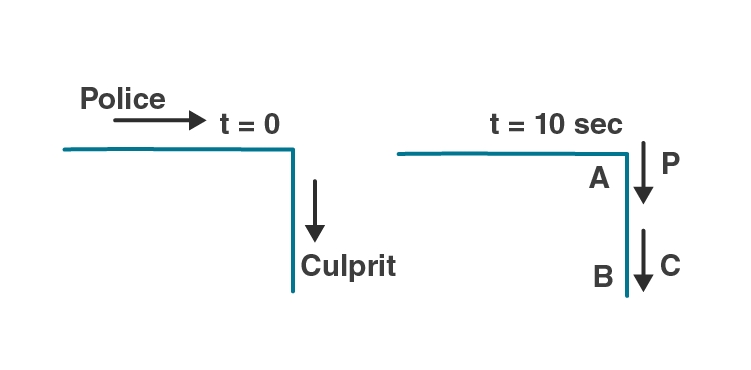In 10 seconds, the culprit reaches point B from point A on motorbike.

So distance covered by the culprit, say s = vt = 20 × 10 = 200 m

It means, Police jeep is 200 m behind the culprit at t = 10 s.

Now, relative velocity between both: Time = s/v = 200/5 = 40 s

Police jeep will move in 40 sec from A to cover distance s,

=> s = vt = 25 x 40 = 1000 m or 1 km away.

Thus, the police jeep will catch up with the bike 1.0 km away from the turning.

Question 22: A car travelling at 60 km/h overtakes another car travelling at 42 km/h. Assuming each car to be 5.0 m long, find the time taken during the overtake and the total road distance used for the overtake.

Solution:

Velocity of the first car, say v1 = 60 km/h = 16.7 m/s

Velocity of the second car, say v2 = 42 km/h = 11.7 m/s

Relative velocity between both the cars = 16.7 – 11.7 = 5 m/s

Consider s be the distance travelled by the first car w.r.t. second car while overtaking,

So, s = 5 + 5 = 10 m

Let t be the time taken while overtaking, t = s/v = 10/5 = 2 s

Now,

Distance covered by first car in 2 sec is,

d = v_1 x t = 16.7 x 2 = 33.4 m

Also, car covered its own length i.e. 5 m.

Thus, the total road distance used for the overtake = 33.4 m + 5 m = 38.4 m

Question 23: A ball is projected vertically upward with a speed of 50 m/s. Find (a) the maximum height, (b) the time to reach the maximum height, (c) the speed at half the maximum height. Take g = 10 m/s2.

Solution:

Initial velocity = u = 50 m/s

Also given, g = a = -10 m/s2

(a) At highest point velocity, v=0.

Using relation, s = (V2-u2)/2a = 125 m

maximum height = 125 m

(b) let t be the time taken to reach at maximum height

t = (V-u)/a = (0-50)/-10 = 5 s

(c)

Half the maximum height = 125/2 = 62.5 m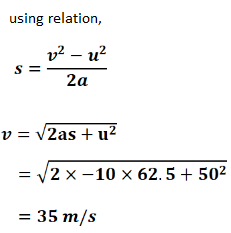Question 24: A ball is dropped from a balloon going up at a speed of 7 m/s. If the balloon was at a height 60 m at the time of dropping the ball, how long will the ball take in reaching the ground?

Solution:

Height of the balloon= s = 60 m

Velocity of the Balloon moving upwards = 7 m/s.

When the ball is dropped, its initial velocity, say u = −7 m/s.

Acceleration due to gravity, a = g = 9.8 m/s2

Using equation: s = ut + 1/2 at2

60 = -7t + 1/2 x 9.8 x t2

=> 4.9t2 – 7t – 60 = 0

or 49t2 – 70t – 600 = 0

Solving above quadratic equation, we get

=>(7t + 20)(7t – 30) = 0

Either 7t + 20 = 0 or 7t – 30 = 0

t = -20/7 or t = 30/7

time cannot be negative, so neglect negative value.

Therefore, ball will take 4.3 sec to reach on the ground.

Question 25: A stone is thrown vertically upward with a speed of 28 m/s. (a) Find the maximum height reached by the stone. (b) Find its velocity one second before it reaches the maximum height. (c) Does the answer of part (b) change if the initial speed is more than 28 m/s such as 40 m/s or 80 m/s?

Solution:

A stone is thrown vertically upward with a speed of 28 m/s.

=> u = 28 m/s

Final velocity = V = 0 (when stone reaches the ground)

Also, Acceleration due to gravity = a = g = 9.8 m/s2

(a)

We know, V2 – u2 = 2as

or s = 40 m

The maximum height reached by the stone is 40 m.

(b)

Using relation, V = u + at

or t = (V-u)/a

or t = 2.85 s

As per the question, we need to find the velocity of the stone one second before it reaches the maximum height.

t’ = 2.85 − 1 = 1.85 s

Again, v’ = u + at’ = 28 − 9.8 × 1.85 = 9.87 m/s

The required velocity is 9.87 m/s

(c) No,

Reason: After one second, the velocity becomes zero for any initial velocity and acceleration (a = − 9.8 m/s2) remains the same.

Question 26: A person sitting on the top of a tall building is dropping balls at regular intervals of one second. Find the positions of the 3rd, 4th and 5th ball when the 6th ball is being dropped.

Solution:

Initial velocity = u = 0 (As person releasing balls at regular intervals of one second.)

Acceleration due to gravity, a = g = 9.8 m/s2

Also, When the 6th ball is dropped, the 5th ball moves for 1 second, the 4th ball moves for 2 seconds and the 3rd ball moves for 3 seconds.

Position of the 3rd ball from the top of the building after t = 3 sec:

S1 = ut + 1/2 at2

= 44.1 m

Position of the 4th ball from the top of the building after t = 2 sec:

S2 = ut + 1/2 at2

= 19.6 m

Position of the 5th ball from the top of the building after t = 1 sec:

S3 = ut + 1/2 at2

= 4.9 m

Question 27: A healthy young man standing at a distance of 7 m from an 11.8 m high building sees a kid slipping from the top floor. With what speed (assumed uniform) should he run to catch the kid at the arms height (1.8 m)?

Solution:

Distance of man from the building = 7 m

Height of the building = 11.8 m

As the kid is slipping, his initial velocity is u which is zero, u = 0.

Acceleration, a = 9.8 m/s2

Let s be the distance before which the kid has to be caught by the young man.

s = 11.8 − 1.8 = 10 m

Using equation, s = ut + 1/2 at2

=> 10 = 0 + 1/2 x 9.8 x t2

=> t = 1.42 sec

Man needs 1.42 sec to reach the bottom of the building to catch the kid.

Again, Velocity = s/t = 7/1.42 = 4.9 m/sec

Man should run with the velocity 4.9 m/sec to catch the kid at the arms.

Question 28: An NCC parade is going at a uniform speed of 6 km/h through a place under a berry tree on which a bird is sitting at a height of 12.1 m. At a particular instant the bird drops a berry. Which cadet (give the distance from the tree at the instant) will receive the berry on his uniform?

Solution:

As per statement,

Distance of the bird from the ground, s = 12.1 m

Speed of the NCC cadets = 6 km/h = 1.66 m/s

Initial velocity of the berry dropped by the bird, u = 0

Acceleration due to gravity, a = g = 9.8 m/s2

Now,

s = ut + 1/2 at2

12.1 = 0 + 1/2 x 9.8 x t2

=> t = 1.57 s

Distance moved by the cadets, say d = v × t = 1.57 × 1.66 = 2.6 m

Therefore, the cadet who is 2.6 m away from tree will receive the berry on his uniform.

Question 29: A ball is dropped from a height. If it takes 0.200 s to cross the last 6.00 m before hitting the ground, find the height from which it was dropped. Take g = 10 m/s2.

Solution:

Distance travelled by the ball in 0.200 sec = 6 m

t = 0.200 s

Distance, s = 6 m

and a = g = 10 m/s2

Now,

s = ut + 1/2 at2

6 = u x 0.2 +1/2 x 10 x 0.04

or u = 29 m/s

Let h be the height from which the ball is dropped.

and u = 0 and v = 29 m/s

Now,

h = (V2-u2)/2a = (29) 2/(2×10) = 42.05 m

Therefore, total height = 42.05 + 6 = 48.05 m

Question 30: A ball is dropped from a height of 5 m onto a sandy floor and penetrates the sand up to 10 cm before coming to rest. Find the retardation of the ball in sand assuming it to be uniform.

Solution:

A ball is dropped from a height of 5 m above the sand level.

The same ball penetrates the sand up to 10 cm before coming to rest.

Initial velocity of the ball, u = 0

and, a = g = 9.8 m/s2

Now,

s = ut + 1/2 at2

5 = 0 + 1/2 x 9.8 x t2

=> t = 1.01 s

The time 1.01 seconds taken by the ball to cover the distance of 5 m.

Again,

Velocity of the ball after 1.01, here u = 0

Using, v = u + at

=> v = 9.8 × 1.01 = 9.89 m/s

Hence, for the motion of the ball in the sand,

initial velocity = 9.89 m/s and

final velocity = 0.

=> s = 10 cm = 0.1 m

Using equation of motion, we have

a = (V2-u2)/2s

a = -490

The sand offers the retardation of 490 m/s2.

Question 31: An elevator is descending with uniform acceleration. To measure the acceleration, a person in the elevator drops a coin at the moment the elevator starts. The coin is 6 ft above the floor of the elevator at the time it is dropped. The person observes that the coin strikes the floor in 1 second. Calculate from these data the acceleration of the elevator.

Solution:

As the elevator descends with uniform acceleration “a” the coin has to move more distance than 6 ft or 1.83 m to strike floor.

Time taken = t = 1 sec and initial velocity = u = 0

Acceleration of coin w.r.t. lift = acceleration of coin w.r.t. earth – acceleration of lift

= g – a = 9.8 – a

Height of the coin from elevator floor = 1.83 m

Using relation, s = ut + 1/2 at2, where a = acceleration of coin w.r.t. lift

1.83 = 0 + 1/2 x (9.8 – a)

a = 6.2 m/s2

Question 32: A ball is thrown horizontally from a point 100 m above the ground with a speed of 20 m/s. Find (a) the time it takes to reach the ground, (b) the horizontal distance it travels before reaching the ground, (c) the velocity (direction and magnitude) with which it strikes the ground.

Solution:

A ball is thrown horizontally from a point 100 m above the ground with a speed of 20 m/s.

It is a case of projectile fired horizontally from a given height.

Here, h = 100 m, g = 9.8 m/s2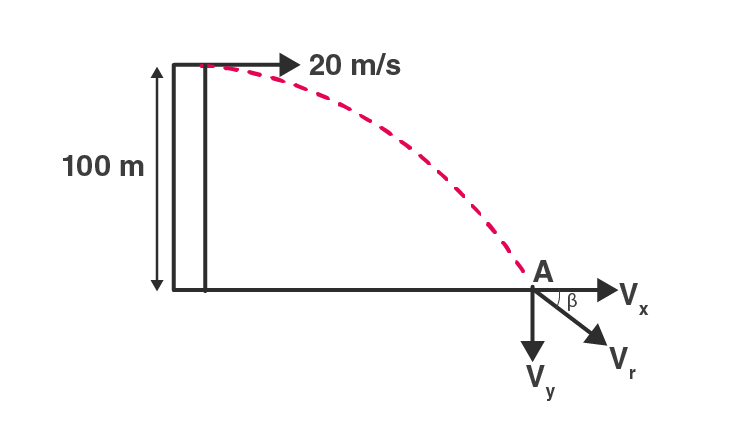a) Time taken to reach the ground = t = √((2h/g) ) = √((2 x 100)/9.8) = 4.51 sec.

b) Horizontal distance travelled by the ball, x = ut = 20 × 4.5 = 90 m.

c) Horizontal velocity remains constant through out the motion of the ball.

From the figure, at point A, Velocity along x-axis Vx= 20 m/s and velocity along y-axis, Vy = u + at = 0 + 9.8 × 4.5 = 44.1 m/s.

Now, Resultant velocity = V = √((44.1) 2 + 202 ) = 48.42 m/s.

Let β be the angle, then

tan β = Vy/Vx = 44.1/20 = 2.205

or β = tan–1 (2.205) = 66°.

Therefore, The ball strikes the ground with a velocity 48.42 m/s at an angle 66° with horizontal.

Question 33: A ball is thrown at a speed of 40 m/s at an angle of 60° with the horizontal. Find (a) the maximum height reached and (b) the range of the ball. Take g = 10 m/s2.

Solution:

A ball is thrown at a speed of 40 m/s at an angle of 60o with the horizontal.

Here,

Initial speed of the ball = u = 40 m/s

Angle of projection of the ball with the horizontal, say θ = 60°

Also, a = g = 10 m/s2

(a) Maximum height reached by ball

H = (u2 sin2θ)/2g = 60 m

(b) Range of the ball.

R = (u2 sin 2θ)/g = 80 √3 m

Question 34: In a soccer practice session the football is kept at the center of the field 40 yards from the 10 ft high goalposts. A goal is attempted by kicking the football at a speed of 64 ft/s at an angle of 45o to the horizontal. Will the ball reach the goal post?

Solution:

initial velocity = u = 40 m/s

angle of projection = θ = 60 o

and a = g = 9.8 m/s2

Now,

Maximum Height, say h = u²sin²θ/2g

h = 60 m

And, Horizontal Range, R = u²sin2θ/g = 80√3 m

Question 35: A popular game in Indian villages is goli which is played with small glass balls called golis. The goli of one player is situated at a distance of 2.0 m from the goli of the second player. This second player has to project his goli by keeping the thumb of the left hand at the place of his goli, holding the goli between his two middle fingers and making the throw. If the projected goli hits the goli of the first player, the second player wins. If the height from which the goli is projected is 19.6 cm from the ground and the goli is to be projected horizontally, with what speed should it be projected so that it directly hits the stationary goli without falling on the ground earlier?

Solution:

The goli of one player is situated at a distance of 2.0 m from the goli of the second player.

Horizontal range = R = 2.0 m

let h be the height from which the goli is projected by the second player = 19.6 cm or 0.196 m.

As goli moves in projectile motion, its acceleration dur to gravity is a = g = 9.8 m/s2

Now,

s = h = u + 1/2 gt2

Here u = 0, as the initial velocity in vertical direction is zero.

=> t = √(2h/g) = 0.2 sec

Let goli is projected with the horizontal velocity U m/s, then the horizontal range, R is

R = Ut

or U = R/t = 2/0/2 = 10 m/s

Hence, second player has to project the goli with a speed of 10 m/s to hit the goli of the first player.

Question 36: Figure (3-E8) shows a 11.7 ft wide ditch with the approach roads at an angle of 15° with the horizontal. With what minimum speed should a motorbike be moving on the road so that it safely crosses the ditch?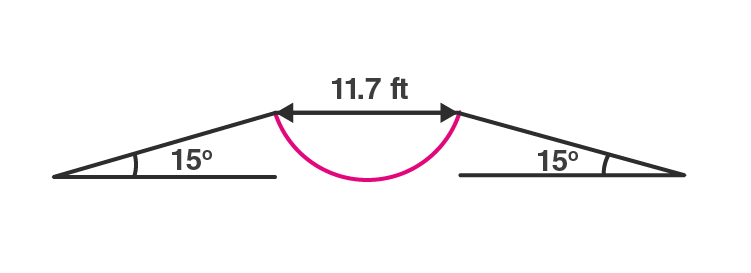Assume that the length of the bike is 5 ft. and it leaves the road when the front part runs out of the approach road.

Solution:

Length of the bike = 5 ft

Width of the ditch = 11.7 ft

The approach road makes an angle of 15° with the horizontal.

Total horizontal range to be covered by the biker to cross the ditch safely,

R = 11.7 + 5 = 16.7 ft

Acceleration due to gravity, a = g = 9.8 m/s = 32.2 ft/s2

Now, the horizontal range, R, is given by

R = (u2 sin 2α)/g

Substituting values, we have u = 32

Therefore, the minimum speed with which the motorbike should be moving is 32 ft/s.

Question 37: A person standing on the top of a cliff 171 ft high has to throw a packet to his friend standing on the ground 228 ft horizontally away. If he throws the packet directly aiming at the friend with a speed of 1.50 ft/s, how short will the packet fall?

Solution:

Let a person at point A is standing at a height 171 feet.

Person A throwing a packet to his friend standing at B, who is 228 feet horizontally away.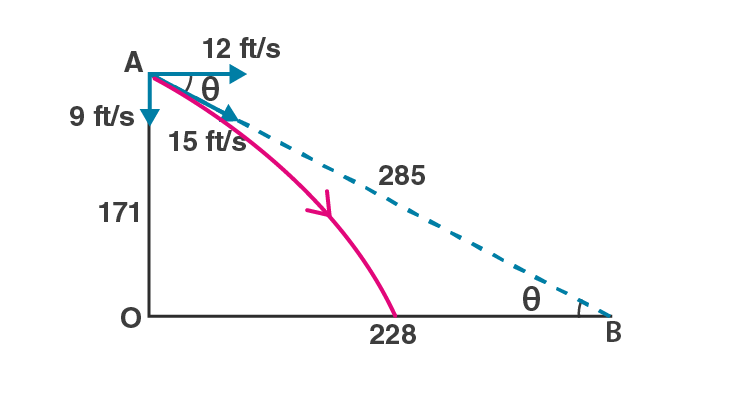Distance between A and B = AB = √(1712+2282) = 85 ft

The person throws the packet directly aiming to his friend at the initial speed (u) of 15.0 ft/s.

Let us find out speed into vertical component and horizontal component.

Vertical component = 15 sinθ = 15×171/285 = 9 ft/s and

Horizontal component = 15 cosθ = 15×228/285 = 12 ft/s

And, acceleration due to gravity is ft/s2. 1 m = 3.281 ft, so g = 9.8×3.281 ft

Now,

S = ut + (1/2)gt2

171 = 9×t+(1/2)×9.8×3.281×t2

= 9×t+16.08×t2

Solving above equation, we have t = 3s

Therefore,

Horizontal distance travelled by the packet = 12×3 = 36 ft.

Distance at which packet will fall short = 288-35.81=192.19 ft.

Question 38: A ball is projected from a point on the floor with a speed of 15 m/s at an angle of 60o with the horizontal. Will it hit a vertical wall 5 m away from the point of projection and perpendicular to the plane of projection without hitting the floor? Will the answer differ if the wall is 22 m away?

Solution:

Initial speed of the ball = u = 15 m/s

Angle of projection with horizontal, θ = 60°

Distance of the wall from the point of projection = 5 ma = g = 9.8 m/s2

Horizontal range for a projectile

R = (u2 sin 2θ)/g = 19.88

The ball will hit the wall 5 m away from the point of projection.

If the wall is 22 m away from the point of projection, the ball will hit the wall as it is not in its horizontal range.

Question 39: Find the average velocity of a projectile between the instants it crosses half the maximum height. It is projected with a speed u at an angle θ with the horizontal.

Solution:

Initial velocity of the projectile = u

Angle of projection = θ

Average velocity = (change in displacement)/time

From the given data, it can be taken as horizontal. So there is no effect of vertical component of the velocity during this displacement.

The projectile moves at a constant velocity ‘u cos θ’ in horizontal direction.

The average velocity of the projectile is u cos θ.

Question 40: A bomb is dropped from a plane flying horizontally with uniform speed. Show that the bomb will explode vertically below the plane. Is the statement true if the plane flies with uniform speed but not horizontally?

Solution:

The bomb follows horizontally projectile with same horizontal velocity as the plane.

Both the plane and bomb are travelling in the same direction.

Distance travelled by the bomb in horizontal direction is “ut”, where u is the speed of the plane and t be the time taken by the bomb to reach the ground.

Also

Distance travelled by the plane in the same time = ut

Hence, the bomb will explode vertically below the plane.

When the plane is flying with a uniform speed but not horizontally:

Again, the bomb will explode vertically below the plane.

because, both will have the same horizontal speed, u cos θ, where u is the initial speed of the plane and the bomb. And the distance travelled by the bomb and the plane will be u cos θ t.

Question 41: A boy standing on a long railroad car throws a ball straight upwards. The car is moving on the horizontal road with an acceleration of 1 m/s and the projection velocity in the vertical direction is 98 m/s. How far behind the boy will the ball fall on the car?

Solution:

Acceleration of the car = 1 m/s2

Projection velocity of the ball in the vertical direction = 9.8 m/s

Angle of projection, say θ = 90o

Both the car and the ball have the same horizontal velocity.

Distance travelled by the ball in horizontal direction, s = ut …(1)

Where u be the initial velocity of the car when the ball is thrown and t = time.

Now,

Distance travelled by the car in horizontal direction:

S = ut + 1/2 at2 ….(2)

Time of flight of projectile:

t = (2u sinθ)/g = 2 sec

[substituting values, u = 9.8, sin 90o = 1, and g = 9.8]

Distance between the accelerated car and the projectile:

From (1) and (2), we have

S – s = 1/2 at2 = 1/2 x 1 x 4 = 2 m

Thus, ball drops 2m behind the boy.

Question 42: A staircase contains three steps each 10 cm high and 20 cm wide (figure 3-E9). What should be the minimum horizontal velocity of a ball rolling off the upper

most plane so as to hit directly the lowest plane?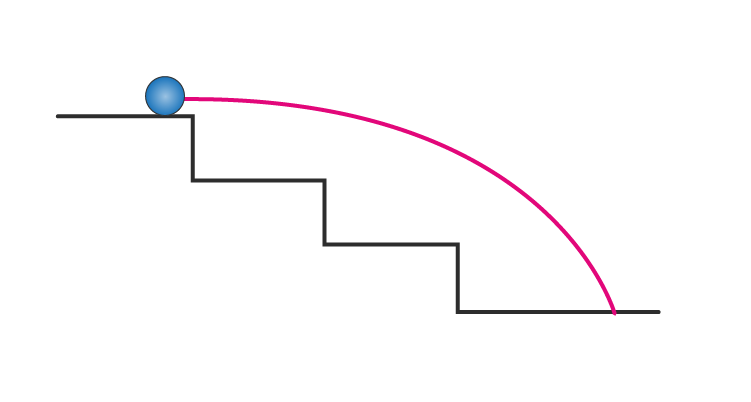Solution:

Dimension of one step:

Height = 10 cm

Width = 20 cm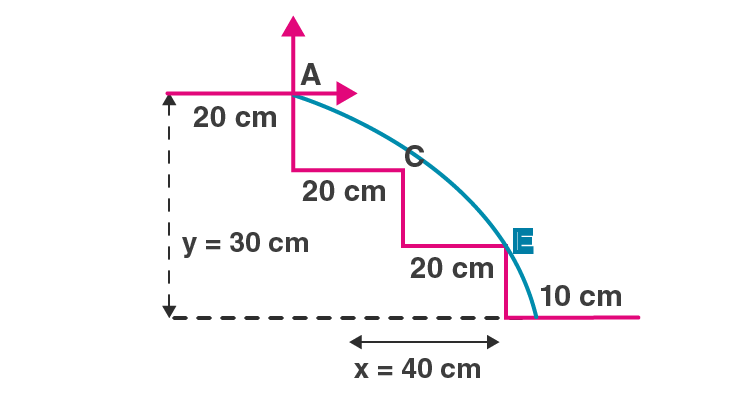form figure,

Total height of the staircase = y = 30 cm

Total width of the staircase = x = 40 cm

To directly hit the lowest one, the ball should just touch E.

Let u = minimum speed of the ball.

Let us consider, A be the origin of reference coordinate.

x = 40 cm, y= -20 cm, g = 10 m/s2 or 1000 cm/s2 and θ = 0o

Now,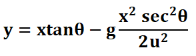=> -20 = -800000/2u2

or u = 2 m/s

The minimum horizontal velocity of the ball is 2 m/s.

Question 43: A person is standing on a truck moving with a constant velocity of 14.7 m/s on a horizontal road. The man throws a ball in such a way that it returns to the truck

after the truck has moved 58.8 m. Find the speed and the angle of projection (a) as seen from the truck, (b) as seen from the road.

Solution:

Velocity of the truck = 14.7 m/s

Distance covered by the truck when ball returns back to the truck= 58.8 m

(a)

Here, time taken by the truck to cover 58.8 m distance = time of the flight of the truck.

T = s/v = 58.8/14.7 = 4 sec

Let us consider, the motion of the ball going upwards at T = 4 s

Time taken to reach the maximum height when v = 0, where v= final velocity

t = T/2 = 2 sec

Again,

V = u – at

where a = g = -9.8 m/s2 and u = initial velocity with which the ball is thrown upwards.

=> u = 19.6 m/s

The motion of ball seems to be a projectile motion, so total time of flight (T) = 4 seconds

Let R be the horizontal range covered by the ball in this time, i.e. R = 58.8 m

We know, the angle of projection, R = u cos αt, where α is the angle of projection.

and u cos α = 14.7 …(1)

Let us work on vertical component of velocity using below equation,

V2 – u2 = 2ay

[substituting values v=0(final velocity), u = 19.6, and a = -9.8]

or y = 19.6 m

Vertical displacement of the ball at t = 2 s:

y = u sin αt – 1/2 gt2

or u sin α = 19.6 …(2)

On dividing (2) by (1), tan α = 1.33

or α = 53o

Again, from (1), u = 25 m/s

Thus, the speed of the ball is 25 m/s and the angle of projection is 53° with horizontal when seen from the road.

Question 44: The benches of a gallery in a cricket stadium are 1 m wide and 1 m high. A batsman strikes the ball at a level one meter above the ground and hits a mammoth sixer. The ball starts at 35 m/s at an angle of 53o with the horizontal. The benches are perpendicular to the plane of motion and the first bench is 110 m from the batsman. On which bench will the ball hit?

Solution:

Width and height of the bench = 1 m

Initial speed of the ball = 35 m/s

Distance of the first bench from the batsman = 110 m.

Angle of projection of the ball = 53 o

or α = 53 o

Let the ball land on the nth bench if batsman strikes the ball 1 m above the ground.

y = n-1 …(1)

and x = 110+n-1 = 110 + y

Again,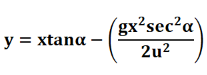By substituting the values, we get

y = 5

(1)=> n-1 = 5 => n = 6

The ball will hit the 6th bench.

Question 45: A man is sitting on the shore of a river. He is in the line of a 1.0 m long boat and is 5.5 m away from the center of the boat. He wishes to throw an apple into the boat. If he can throw the apple only with a speed of 10 m/s, find the minimum and maximum angles of projection for successful shot. Assume that the point of projection and the edge of the boat are in the same horizontal level.

Solution:

Initial speed of throwing the apple by the man= u = 10 m/s

Length of the boat = 1.0 m

Distance between the man and the center of the boat (R) = 5.5 m

Acceleration due to gravity =g = 10 m/s2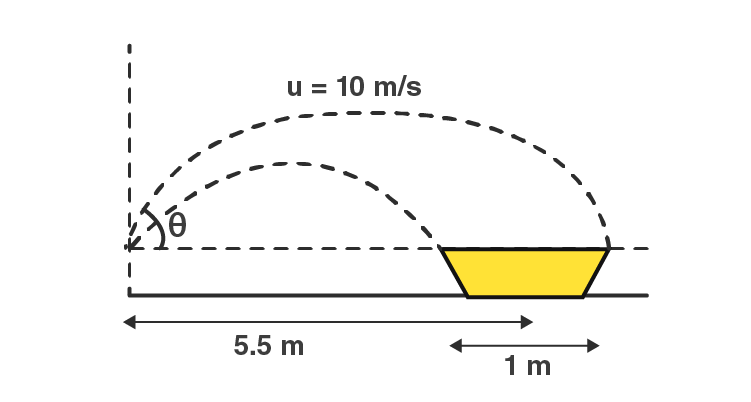The horizontal range:

R = (u2 sin2θ)/g

by substituting the values, we get

sin2θ = 1/2

or θ = 15 o or 75o

For the end point of the boat:

Horizontal range (R) = 6 m

for the end point of the boat, we have:

Horizontal range (R) = 6 m

R = (u2 sin2θ)/g

Here θ = 18 o or 71 o

For a successful shot, the angle of projection with initial speed 10 m/s may vary from 15° to 18° or from 71° to 75°.

Question 46: A river 400 m wide is flowing at a rate of 2.0 m/s. A boat is sailing at a velocity of 10 m/s with respect to the water, in a direction perpendicular to the river.

(a) Find the time taken by the boat to reach the opposite bank. (b) How far from the point directly opposite to the starting point does the boat reach the opposite bank?

Solution:

Distance between the opposite shore of the river or width of the river = 400 m

Boat is sailing at the rate of 10 m/s.

Rate of flow of the river = 2.0 m/s

let v be the resultant velocity of the boat when vertical component of velocity 10 m/s takes the boat to the opposite shore.

Time taken = 400/10 = 40 sec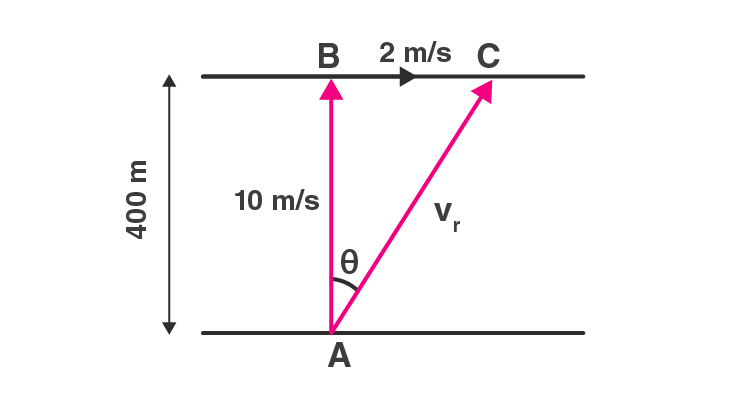Now,

tan θ = 2/10 = 1/5

When boat reach at point C

From the figure, in triangle ABC

tan θ = BC/AB = 1/5

or BC = 400/5 = 80 m

Also, |v| = √(102+22) = 10.2 m/s

Let α be the angle made by the boat sailing with respect to the direction of flow.

tan α = 10/2 or α = 78.7o

The distance boat has to travel to reach the opposite shore = 400/sinα = 407.9 m

(a)

Time taken by the boat to reach the opposite bank = 407.9/10.2 = 40 sec

(b)

Distance = √(407.92-4002) = 79.9 m or 80 m (approx)

Question 47: A swimmer wishes to cross a 500 m wide river flowing at 5 km/h. His speed with respect to water is 3 km/h. (a) If he heads in a direction making an angle θ with the flow, find the time he takes to cross the river. (b) Find the shortest possible time to cross the river.

Solution:

Width of the river = 500 m

Swimmer’s speed with respect to water = 3 km/h

Rate of flow of the river = 5 km/h

The swimmer heads in a direction making an angle θ with the flow.

So, 3 sinθ is the vertical component of velocity which takes swimmer to the opposite side of the river.

Vertical component of velocity = 3sinθ km/h

Distance to be travelled = 0.5 km

(a)

Time = 0.5h/3sinθ = (500×6)/5sinθ) = 600/sinθ sec or 10/sinθ min

(b)

Here θ = 90o

Time = 0.5h/3sinθ = (500×6)/5sin90 o = 600 sec

Therefore, shortest possible time to cross the river is 10 mins.